# Convert a Binary Search Tree into a min-heap

In this article, we are going to see how we can convert a Binary Search Tree into min-heap?
Submitted by Radib Kar, on October 13, 2020

Prerequisite: Introduction to Heap

In this article, we are going to see how to convert a given binary search tree into a min-heap? In our article on introduction to the heap data structure, we have already seen that a min-heap is a complete binary tree where each parent has a smaller key than its children's. Now, to convert a BST into a min-heap, let's understand what the difference between a Binary Search Tree and a min-heap is. So, in case of a BST, a parent has its immediate left child smaller than this and its immediate right child is greater than the parent. But in the case of min-heap, the parent has to be the smaller. So, it's clear that we need to convert the BST to something intermediate before converting it into a min HEAP.

We can either convert the BST into a sorted array or we can just convert into a sorted linked list. In this article, we have created a sorted array out of the Binary Search Tree. Then we can convert the sorted array to a min-heap.

1. Converting BST to a sorted array
The inorder traversal of the BST will produce a sorted vector/array. WE will pass a vector in the inorder traversal and will keep pushing the elements into the vector. Below is the code snippet to do that
```void inorder(TreeNode* root,vector &store){
if(!root)
return;
inorder(root->left, store);
store.push_back(root->val);
inorder(root->right, store);
}
```
2. Now to create heap from this sorted array we need to ensure that each parent needs to be smaller than its child. That's possible if we keep inserting the elements one by one level wise.

To illustrate this, let's have a BST first.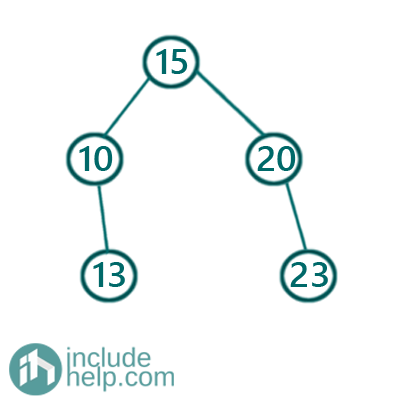Then after converting to a sorted array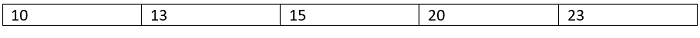Now, we need to insert the nodes level wise and have to create a complete tree. So, for each level, the insertion will be left to right. SO the left parents will have children inserted first.

Let's see the visual illustration of how we insert elements into the tree(which is going to be the heap)

So 10 will be the root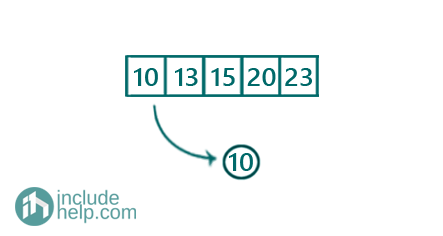Then 13 will be the immediate left child of 10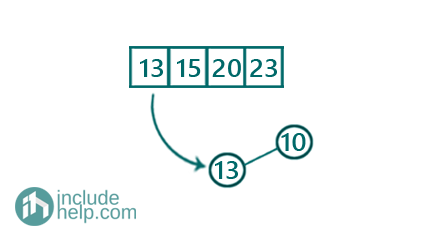15 will be the immediate right child of 10(level wise insertion from left to right)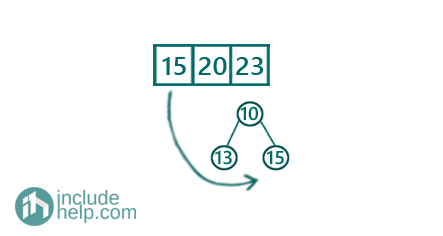Level 2 ends here and now, it will be the start of level 3 and we will insert the element 20 as the left child of 13(leftmost first).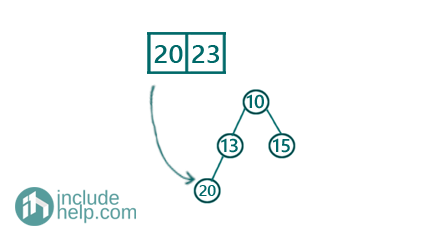Then we will insert the final one 23.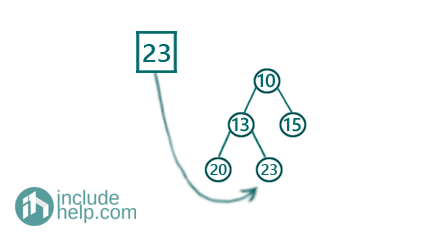Since it was a sorted array, level-wise insertion automatically took care of the fact that parent will be smaller than its children and as we inserted level-wise left to right that ensured it will be a complete tree. Overall we converted the binary search tree into a min-heap.

Below is the implementation

```#include <bits/stdc++.h>
using namespace std;

// tree node is defined
class TreeNode {
public:
int val;
TreeNode* left;
TreeNode* right;
TreeNode(int data)
{
val = data;
left = NULL;
right = NULL;
}
};

//level order traversal for BST
void levelorder(TreeNode* root)
{
if (!root)
return;

queue<TreeNode*> q;
q.push(root);
q.push(NULL);

int count = 1;
cout << "Start of level: " << count << endl;
while (!q.empty()) {
TreeNode* temp = q.front();
q.pop();
if (temp == NULL) {
if (!q.empty()) {
q.push(NULL);
cout << "\nEnd of level: " << count << endl;
count++;
cout << "Start of level: " << count << endl;
}
else {
cout << "\nEnd of level: " << count << endl;
}
}
else {
cout << temp->val << " ";
if (temp->left)
q.push(temp->left);
if (temp->right)
q.push(temp->right);
}
}
}

//storing the inorder traversal
void inorder(TreeNode* root, vector<int>& store)
{
if (!root)
return;
inorder(root->left, store);
store.push_back(root->val);
inorder(root->right, store);
}

//convert to min heap from BST
TreeNode* convertToMinHeap(vector<int>& store)
{
int index = 0;
TreeNode* root = new TreeNode(store[index++]);

queue<TreeNode*> q;
q.push(root);
//insert level wise
while (!q.empty()) {
TreeNode* temp = q.front();
q.pop();
if (index == store.size())
return root;
//first insert as left child
temp->left = new TreeNode(store[index++]);
q.push(temp->left);
if (index == store.size())
return root;
//then insert as right child
temp->right = new TreeNode(store[index++]);
q.push(temp->right);
}
return root;
}

int main()
{
//below is the unbalanced BST as per example
TreeNode* root = new TreeNode(15);
root->left = new TreeNode(10);
root->right = new TreeNode(20);
root->left->right = new TreeNode(13);
root->right->right = new TreeNode(23);
cout << "Level order traversal of the BST:\n";
levelorder(root);
cout << "Converting the BST into min heap\n";
vector<int> store;
inorder(root, store);

TreeNode* heap = convertToMinHeap(store);
cout << "Converted into a min heap...\n";
cout << "Level order traversal of the min heap is :\n";
levelorder(heap);

return 0;
}
```

Output:

```Level order traversal of the BST:
Start of level: 1
15
End of level: 1
Start of level: 2
10 20
End of level: 2
Start of level: 3
13 23
End of level: 3
Converting the BST into min-heap
Converted into a min-heap...
Level order traversal of the min-heap is :
Start of level: 1
10
End of level: 1
Start of level: 2
13 15
End of level: 2
Start of level: 3
20 23
End of level: 3
```

You might be wondering to thinking that here we have represented the min-heap as a tree instead of an array. But that's indeed a representation as a min-heap is a complete tree. Here the tree representation suited more than the array representation.

Top MCQs

Languages: » C » C++ » C++ STL » Java » Data Structure » C#.Net » Android » Kotlin » SQL
Web Technologies: » PHP » Python » JavaScript » CSS » Ajax » Node.js » Web programming/HTML
Solved programs: » C » C++ » DS » Java » C#
Aptitude que. & ans.: » C » C++ » Java » DBMS
Interview que. & ans.: » C » Embedded C » Java » SEO » HR
CS Subjects: » CS Basics » O.S. » Networks » DBMS » Embedded Systems » Cloud Computing
» Machine learning » CS Organizations » Linux » DOS
More: » Articles » Puzzles » News/Updates